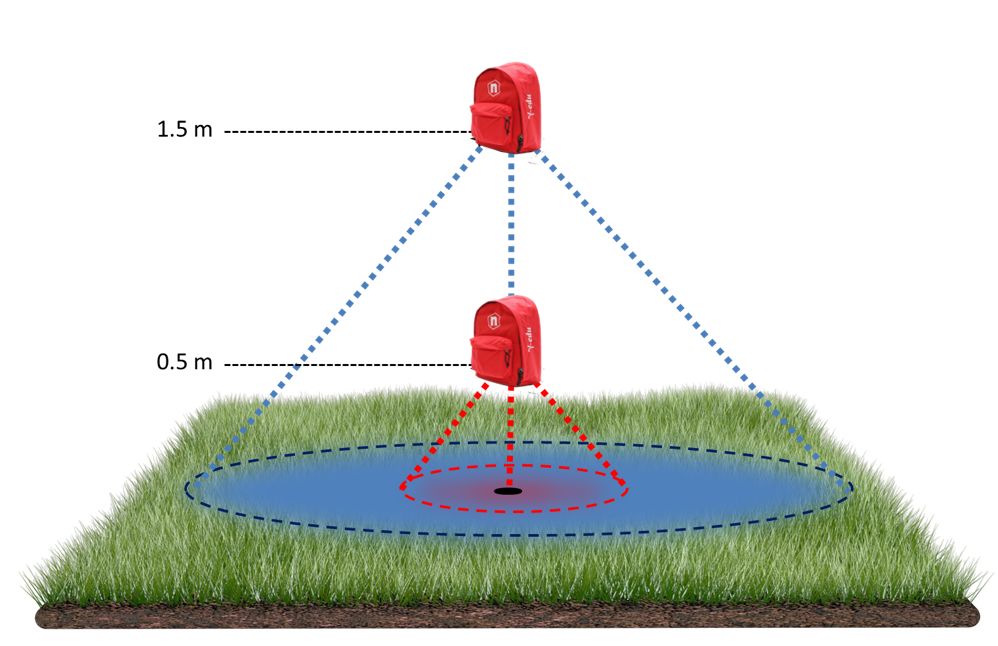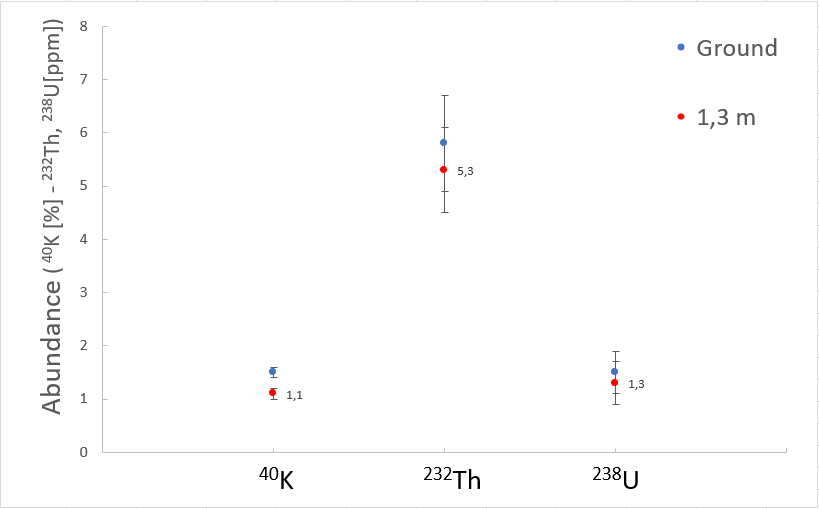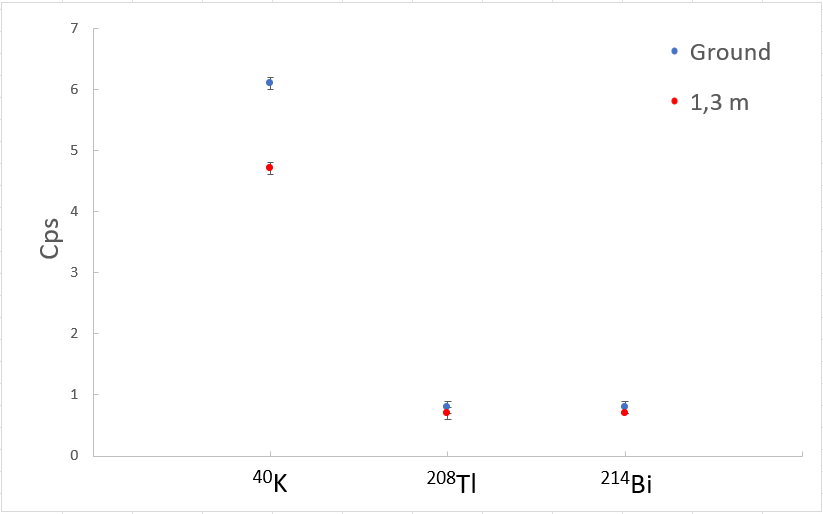Difficult Execution Time Data Analysis Radioactive SourcesYes No

#### Purpose of the experiment:

The main goal of the experiment is to understand how the measurement of the γ environmental radiation can be affect by the distance of the point of measurement.

#### Fundamentals:

The linear attenuation coefficient of gamma radiation µ represents the inverse of the distance at which the number of photons is reduced by a factor 1/e, as can be inferred by the following equation: N = N0eµx , where µ in cm1. This Equation is the key for understanding the lateral horizon of in-situ gamma ray spectroscopy. The horizontal field of view of a gamma-ray detector expresses the relative contribution to the total signal that is produced within a given radial distance from the detector vertical axis. The lateral horizon depends on the height of the detector: for instance, a spectrometer that was placed at ground level detect gammas coming from the first 25 cm of depth and it receives 90% of the signal from a radius of ~0.5 m. At a height of 0.5 m, 95% of the signal come from a radius of ~8 m and the maximum percentage contribution to the flux comes from the concentric hollow cylinder of soil having a ~0.6 m radius centered at the detector vertical axis. When it’s carried on the shoulders of the operator (1.5 m of height) the signal reaches ~95% at ~20 m and the maximum percentage contribution to the flux comes from the concentric hollow cylinder with a radius of ~1.2 m.

#### Requirements:

No other tool is needed.#### Carrying out the experiment:

Power on the γstream inside the red backpack. Power on the tablet and associate the two devices via Bluetooth.
Take care that the γstream internal battery is charged, otherwise use the external power system.
Start the experiment by placing the backpack on the floor/soil. Set the acquisition time to about 5 minutes and see the results. If the statistic is not enough increasing the acquisition time.
Repeat the measurements at different heights/distances from soil and pay attention to perform the measurements in the same conditions exactly.

#### Results:

The measurements results show a gamma detection decrease as a function of the soil distance.a)                                                                                                    b)
Result of the in-situ-y-ray measurements that were performed at different heights: the counts per second go three isotopes (a) and the abundance (b).